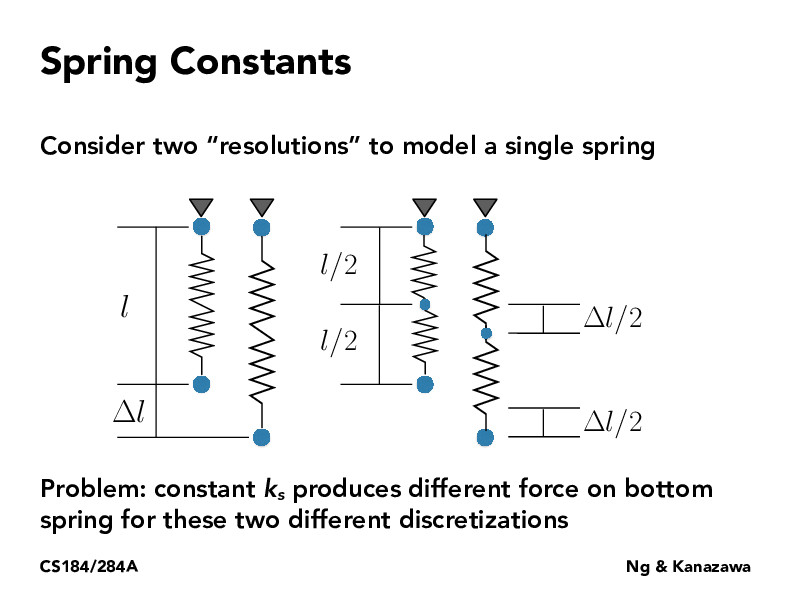Lecture 18: Introduction to Physical Simulation (16)zhangyifei-chelsea

I am not sure why the force on bottom spring will be different with different discretizations. To my mind, for the left case, the force is $k_s(\Delta l)$, and for the right case, the accumulated force is $k_s(\Delta l / 2) + k_s(\Delta l / 2) = k_s(\Delta l)$... So I think they are still the same...SainanChen

I guess in the previous slides we assume the lengths of springs are the same. Actually the force should be proportional to the proportional change of length, and the forces here should be the same. I guess here it means if we still use the previous consumption, which is not true here, the result would be unreasonable.sutkarsh

@zhangyifei-chelsea @SainanChen Here's another way to think about this: if force F is applied to the spring system on the right, each spring experiences that force, and stretches proportionally ($\delta l$), resulting in a total $2\delta l$ stretch. The only way to keep the stretch the same is to apply less foce.ronthalanki

@sutkarsh Why does each spring (in the system on the right) experience the force F? F is only directly applied to the bottom spring and the bottom spring will apply some force F_2 to the upper spring?briana-jin-zhang

I think this is related to how Kanazawa talks about in the next slide what we care about is the percentage of change in length rather than the absolute change in length. I think right now we are treating the springs as different entities which both stretch by $\delta l / 2$, which causes a force of $k_s \delta l / 2$ for the bottom spring instead of $k_s \delta l$Leo-godel

For the left bottom, the force applied is ks * deltaL. However, for the right bottom, the force applied is ks * deltaL / 2. I think one way to solve this problem is to redefine the ks, making it relative to the length or use the relative percentage deformation rather than the absolute value.briana-jin-zhang

Rewatching lecture, I feel like the issue can be more clearly seen as if you try to calculate the force in the springs with the simple spring model for example, you end up with half the force since f = k_d (b - a) where b-a is halved in the bottom spring. But you actually want the force to be equal.

You must be enrolled in the course to comment